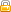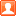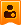[使用说明] 虫子机器人群管娱乐菜单[复制链接]发表于 2018-2-25 18:47:30 | 显示全部楼层 |阅读模式

马上注册，结交更多好友，享用更多功能，让你轻松玩转社区。

x

1. 功能菜单

1. 群管菜单

1. 踢@TA楼主| 发表于 2019-3-30 09:43:17 | 显示全部楼层
 【群主命令】管理员不可用 开启某个群的群管=开启群管复制代码 关闭某个群的群管=关闭群管复制代码 打开欢迎新人开关（新人进群在群里发欢迎语）=开启群欢迎复制代码 关闭欢迎新人开关（新人进群在群里发欢迎语）=关闭群欢迎复制代码 开启群私聊（新人进群私聊发欢迎语）=开启群私聊复制代码 关闭群私聊（新人进群私聊发欢迎语）=关闭群私聊复制代码 开启群退出（新人退群时的提示）=开启群退出复制代码 关闭群退出（新人退群时的提示）=关闭群退出复制代码 开启加管理（群里添加管理时的提示）=开启加管理复制代码 关闭加管理（群里添加管理时的提示）=关闭加管理复制代码 开启删管理（群里撤管理时的提示）=开启删管理复制代码 关闭删管理（群里撤管理时的提示）=关闭删管理复制代码 开启群被踢（群成员被踢时的提示）=开启群被踢复制代码 关闭群被踢（群成员被踢时的提示）=关闭群被踢复制代码 设置群欢迎提示（设置进群时机器人发的群欢迎内容）=设置群欢迎提示复制代码 设置群退出提示（设置群成员退群时机器人发的内容）=设置群退出提示复制代码 设置被踢出提示（设置群成员被踢时机器人发的内容）=设置被踢出提示复制代码 设置加管理提示（设置群成员被设为管理员时机器人发的内容）=设置加管理提示复制代码 设置删管理提示（设置群管理被撤职时机器人发的内容）=设置删管理提示复制代码 设置进群私聊提示（设置进群时机器人私聊群员发的内容）=设置进群私聊提示 复制代码 开启退群拉黑（开启后群成员退群会被加入黑名单）=开启退群拉黑复制代码 关闭退群拉黑（关闭后群成员退群不会被加入黑名单）=关闭退群拉黑复制代码 开启踢出拉黑（开启后群成员被踢出会被加入黑名单）=开启踢出拉黑复制代码 关闭踢出拉黑（关闭后群成员被踢出不会被加入黑名单）=关闭踢出拉黑复制代码 开启踢出拉黑提示（开启后群成员被踢出自动加黑名单时会提示）=开启踢出拉黑提示复制代码 关闭踢出拉黑提示（关闭后群成员被踢出自动加黑名单时不会提示）=关闭踢出拉黑提示复制代码 开启退群拉黑提示（开启后群成员退群自动加黑名单时会提示）=开启退群拉黑提示复制代码 关闭退群拉黑提示（关闭后群成员退群自动加黑名单时不会提示）=关闭退群拉黑提示复制代码 添加敏感词（防广告和炸群，触发自动撤回+你设置的处理方式）=添加敏感词复制代码格式：添加敏感词+内容【处理方式】 例如：添加敏感词呵呵哒【2，30】->代表，如果群成员内容包含呵呵哒，将会被警告且禁言30分钟并撤回本条消息 类型：1、警告 2、警告且禁言 3 禁言 4、踢出 如果希望直接踢出，则是：添加敏感词呵呵哒【4】 删除敏感词（删除加入的敏感词）=删除敏感词复制代码格式：删除敏感词+内容，例如：删除敏感词呵呵哒 开启敏感词检测（开启后会检测并处理设置好的敏感词）=开启敏感词检测复制代码 关闭敏感词检测（关闭后不会检测）=关闭敏感词检测复制代码 开启进群改名（进群会自动按格式改名）=开启进群改名复制代码 关闭进群改名（进群不会自动按格式改名）=关闭进群改名复制代码 开启自由改名（群成员可在群用命令自由改名）=开启自由改名复制代码 关闭自由改名（群成员不可在群用命令自由改名）=关闭自由改名复制代码 设置男名片的格式=设置男名片复制代码 设置女名片的格式=设置女名片复制代码 设置人妖名片的格式=设置人妖名片复制代码 艾特全体成员=@全体复制代码 查询串群（查询某个人是否同时在两个群）=查询串群复制代码正确指令：查询串群第一个群，第二个群，例如：查询串群523869830，579851282 踢出串群（如某人同时在两个群，则从第二个群踢出）=踢出串群复制代码正确指令：踢出串群第一个群，第二个群，例如：踢出串群523869830，579851282 检测群成员多久未发言=检测发言时间+天数复制代码例如：检测发言时间90 踢出进群从未发言过的人=踢出未发言人复制代码 踢出多少天没有发言过的人=踢出发言复制代码例如：踢出发言90 开启词库(词库)=开启词库复制代码 关闭词库(词库)=关闭词库复制代码 精确问(词库)=精确问复制代码 模糊问(词库)=模糊问复制代码 添加词库(词库)=添加词库复制代码 删除(词库)=删除复制代码 删除词库(词库)=删除词库复制代码 查询id(词库)=查询id复制代码 查(词库)=查复制代码 查询词库(词库)=查询词库复制代码 批量加黑=批量加黑复制代码 清空白名单=清空白名单复制代码 清空黑名单=清空黑名单复制代码 一键踢出黑名单=一键踢出黑名单复制代码 设置同意进群=设置同意进群复制代码 设置拒绝进群=设置拒绝进群复制代码 设置忽略进群=设置忽略进群复制代码 设置不操作进群=设置不操作进群复制代码 设置拒绝加群理由=设置拒绝加群理由复制代码 设置进群等级=设置进群等级复制代码 开启限制等级进群=开启限制等级进群复制代码 关闭限制等级进群=关闭限制等级进群复制代码 开启个签检测=开启个签检测复制代码 关闭个签检测=关闭个签检测复制代码 开启昵称检测=开启昵称检测复制代码 关闭昵称检测=关闭昵称检测复制代码 开启进群发言=开启进群发言复制代码 关闭进群发言=关闭进群发言复制代码 添加管理=添加管理复制代码 删除管理=删除管理复制代码 开启危险人员检测=开启危险人员检测复制代码 关闭危险人员检测=关闭危险人员检测复制代码 开启投票(投票踢人)=开启投票复制代码 关闭投票(投票踢人)=关闭投票复制代码 开启刷屏检测(刷屏检测)=开启刷屏检测复制代码 关闭刷屏检测(刷屏检测)=关闭刷屏检测复制代码 开启刷屏撤回(刷屏检测)=开启刷屏撤回复制代码 关闭刷屏撤回(刷屏检测)=关闭刷屏撤回复制代码 人数控制/(进群审核)=人数控制复制代码 开启人数控制(进群审核)=开启人数控制复制代码 关闭人数控制(进群审核)=关闭人数控制复制代码 人数控制规则活跃(进群审核)=人数控制规则活跃复制代码 人数控制规则加群(进群审核)=人数控制规则加群复制代码 开启进群附言检测(进群审核)=开启进群附言检测复制代码 关闭进群附言检测(进群审核)=关闭进群附言检测复制代码 查看进群附言理由(进群审核)=查看进群附言理由复制代码 设置进群附言理由/(进群审核)=设置进群附言理由复制代码 开启连续图片(刷屏检测)=开启连续图片复制代码 关闭连续图片(刷屏检测)=关闭连续图片复制代码 设置刷屏连续图片/(刷屏检测)=设置刷屏连续图片复制代码 开启连续消息(刷屏检测)=开启连续消息复制代码 关闭连续消息(刷屏检测)=关闭连续消息复制代码 设置刷屏连续消息/(刷屏检测)=设置刷屏连续消息复制代码 开启文字超限(刷屏检测)=开启文字超限复制代码 关闭文字超限(刷屏检测)=关闭文字超限复制代码 设置刷屏文字超限/(刷屏检测)=设置刷屏文字超限复制代码 开启相同文本(刷屏检测)=开启相同文本复制代码 关闭相同文本(刷屏检测)=关闭相同文本复制代码 设置刷屏相同文本/(刷屏检测)=设置刷屏相同文本复制代码 开启文字超行(刷屏检测)=开启文字超行复制代码 关闭文字超行(刷屏检测)=关闭文字超行复制代码 设置刷屏文字超行/(刷屏检测)=设置刷屏文字超行复制代码 开启图片超限(刷屏检测)=开启图片超限复制代码 关闭图片超限(刷屏检测)=关闭图片超限复制代码 设置刷屏图片超限/(刷屏检测)=设置刷屏图片超限复制代码 设置投票权限/(投票)=设置投票权限复制代码 开启投票类型1~4之间(投票踢人)=开启投票类型复制代码 关闭投票类型1~4之间(投票踢人)=关闭投票类型复制代码 开启投票条件1~4之间(投票踢人)=开启投票条件复制代码 关闭投票条件1~4之间(投票踢人)=关闭投票条件复制代码 设置投票一  代表 群等级(投票踢人)=设置投票一复制代码 设置投票二  代表 Q等级(投票踢人)=设置投票二复制代码 设置投票三  代表 Q年龄(投票踢人)=设置投票三复制代码 设置投票四  代表 性别(投票踢人)=设置投票四复制代码 设置禁言票数(投票踢人)=设置禁言票数复制代码 设置禁言时间(投票踢人)=设置禁言时间复制代码 设置踢出票数(投票踢人)=设置踢出票数复制代码 设置每日票数(投票踢人)=设置每日票数复制代码 设置截止时间(投票踢人)=设置截止时间复制代码 开启邀请统计(邀请统计)=开启邀请统计复制代码 关闭邀请统计(邀请统计)=关闭邀请统计复制代码 开启审核发言(邀请统计)=开启审核发言复制代码 关闭审核发言(邀请统计)=关闭审核发言复制代码 开启审核退群(邀请统计)=开启审核退群复制代码 关闭审核退群(邀请统计)=关闭审核退群复制代码 开启审核踢出(邀请统计)=开启审核踢出复制代码 关闭审核踢出(邀请统计)=关闭审核踢出复制代码 开启审核管理(邀请统计)=开启审核管理复制代码 关闭审核管理(邀请统计)=关闭审核管理复制代码 开启邀请提示语(邀请统计)=开启邀请提示语复制代码 关闭邀请提示语(邀请统计)=关闭邀请提示语复制代码 开启查询提示语(邀请统计)=开启查询提示语复制代码 关闭查询提示语(邀请统计)=关闭查询提示语复制代码 开启被查提示语(邀请统计)=开启被查提示语复制代码 关闭被查提示语(邀请统计)=关闭被查提示语复制代码 设置邀请提示语(邀请统计)=设置邀请提示语复制代码 设置查询提示语(邀请统计)=设置查询提示语复制代码 设置被查提示语(邀请统计)=设置被查提示语复制代码 设置审核发言(邀请统计)=设置审核发言复制代码 设置审核退群(邀请统计)=设置审核退群复制代码 设置审核踢出(邀请统计)=设置审核踢出复制代码 设置邀请奖励(邀请统计)=设置邀请奖励复制代码 开启静默处理(敏感词)=开启静默处理复制代码 关闭静默处理(敏感词)=关闭静默处理复制代码 开启二维处理(敏感词)=开启二维处理复制代码 关闭二维处理(敏感词)=关闭二维处理复制代码 开启撤回处理(敏感词)=开启撤回处理复制代码 关闭撤回处理(敏感词)=关闭撤回处理复制代码 开启禁言处理(敏感词)=开启禁言处理复制代码 关闭禁言处理(敏感词)=关闭禁言处理复制代码 开启踢出处理(敏感词)=开启踢出处理复制代码 关闭踢出处理(敏感词)=关闭踢出处理复制代码 开启图片处理(敏感词)=开启图片处理复制代码 关闭图片处理(敏感词)=关闭图片处理复制代码 设置敏感词要求如3.5.7(敏感词)=设置敏感词要求复制代码 开启文件监控(文件监控)=开启文件监控复制代码 关闭文件监控(文件监控)=关闭文件监控复制代码 开启监控群主(文件监控)=开启监控群主复制代码 关闭监控群主(文件监控)=关闭监控群主复制代码 开启监控管理(文件监控)=开启监控管理复制代码 关闭监控管理(文件监控)=关闭监控管理复制代码 开启监控群员(文件监控)=开启监控群员复制代码 关闭监控群员(文件监控)=关闭监控群员复制代码 开启上传提示(文件监控)=开启上传提示复制代码 关闭上传提示(文件监控)=关闭上传提示复制代码 开启上传限制(文件监控)=开启上传限制复制代码 关闭上传限制(文件监控)=关闭上传限制复制代码 设置文件大小(文件监控)=设置文件大小复制代码 设置允许格式(文件监控)=设置允许格式复制代码 修改允许格式(文件监控)=修改允许格式复制代码 设置监控词(文件监控)=设置监控词复制代码 修改监控词(文件监控)=修改监控词复制代码 设置上传提示语(文件监控)=设置上传提示语复制代码 设置监控白名单(文件监控)=设置监控白名单复制代码 设置刷屏禁言时间(刷屏检测)=设置刷屏禁言时间复制代码 设置刷屏禁言次数(刷屏检测)=设置刷屏禁言次数复制代码楼主| 发表于 2019-3-30 09:44:06 | 显示全部楼层
 管理员和群主命令（管理员和群主可用） 查警告次数=查警告次数复制代码 清除警告次数=清除警告次数复制代码 改名=改名复制代码 禁言=禁言复制代码 踢出=踢复制代码 解除禁言=解禁复制代码 全体禁言=全体禁言复制代码 全体解禁=全体解禁复制代码 清屏=清屏复制代码 公告=公告复制代码 发公告=发公告复制代码 清除刷屏次数=清除刷屏次数复制代码 查黑=查黑复制代码 查白=查白复制代码 加黑=加黑复制代码 删黑=删黑复制代码 加白=加白复制代码 删白=删白复制代码 白名单数量=白名单数量复制代码 黑名单数量=黑名单数量复制代码 管理员列表=管理员列表复制代码 群管菜单=群管菜单复制代码 警告某QQ=警告复制代码 去掉某qq的一次警告次数=去警告复制代码 发作业通知功能=发作业复制代码 邀请统计(邀请统计)=邀请统计复制代码 邀请查询 私聊情况下 群号+Q号(邀请统计)=邀请查询复制代码 投票结束=投票结束复制代码楼主| 发表于 2019-3-30 09:44:19 | 显示全部楼层
 普通群员以上可用（群友群主管理员都可用） 投票禁言=投票禁言复制代码 投票踢出=投票踢出复制代码楼主| 发表于 2019-3-30 09:34:34 | 显示全部楼层
 使用说明 一.首次邀请机器人加群，请加群获取机器人授权。（QQ群：523869830） 二.授权以后邀请机器人进群，设置机器人为群管理员，该群群主和管理员自动有机器人控制权限。 基础说明[必做必看] 1.开启指令：开启群管，停用指令：关闭群管，群主可以操作。 2.所有的指令不需要艾特机器人，可以直接发送。 3. 请勿将机器人禁言，必须将机器人设置为群管理员。 4..所有的设置都可以通过群内直接发送进行设置（有些命令仅限群主使用） 特色功能 自动警告禁言和自动撤回   此项功能由机器自动完成，无需指令！ 当群成员触发敏感词，机器人会自动撤回该群成员消息并且自动警告禁言一次。当警告达到5次时，自动踢出被警告者。（触发的敏感词以及触发后是否自动撤回/禁言时间/是否自动禁言或自动踢出该成员，可由群主在群内通过指令设置） 联系方式 如果有想租的可以联系我，也有纯群管机器人。 群聊机器人体验中心：523869830（QQ群） 欢迎打扰。楼主| 发表于 2019-3-30 09:41:36 | 显示全部楼层楼主| 发表于 2019-3-30 09:41:53 | 显示全部楼层楼主| 发表于 2019-3-30 09:49:11 | 显示全部楼层 回复 支持 反对